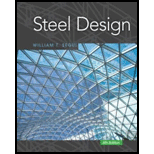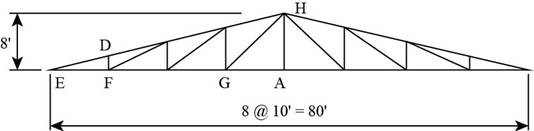# Design the tension members of the roof truss shown in Figure P3.8-4. Use, double-angle shapes throughout and assume 3 8 -inch-thick gusset plates and welded connections. Assume a shear lag factor of U = 0.85. The trusses are spaced 25 feet. Use A572 Grade 50 steel and design for the following loads. Metal deck: 4 psf of roof surface Build-up roof: 12 psf of roof surface Purlins: 6 psf of roof surface (estimated) Snow: 18 psf of horizontal projection Truss weight: 5 psf of horizontal projection (estimated) a . Use LRFD. b . Use ASD.### Steel Design (Activate Learning wi...

6th Edition
Segui + 1 other
Publisher: Cengage Learning
ISBN: 9781337094740

#### Solutions

Chapter
Section### Steel Design (Activate Learning wi...

6th Edition
Segui + 1 other
Publisher: Cengage Learning
ISBN: 9781337094740
Chapter 3, Problem 3.8.4P
Textbook Problem
810 views

## Design the tension members of the roof truss shown in Figure P3.8-4. Use, double-angle shapes throughout and assume 3 8 -inch-thick gusset plates and welded connections. Assume a shear lag factor of U = 0.85. The trusses are spaced 25 feet. Use A572 Grade 50 steel and design for the following loads.Metal deck: 4 psf of roof surfaceBuild-up roof: 12 psf of roof surfacePurlins: 6 psf of roof surface (estimated)Snow: 18 psf of horizontal projectionTruss weight: 5 psf of horizontal projection (estimated)a. Use LRFD.b. Use ASD.To determine

(a)

The double angle section for a tension member of the roof truss using Load And Resistance Factor Design (LRFD) method.

### Explanation of Solution

Given:

Metal deck load is 4psf of roof surface.

Build up roof load is 12psf.

Purlins load is 6psf of roof surface.

Snow load is 18psf of horizontal projection.

Truss load is 5psf of horizontal projection.

The trusses are spaced at 25ft.

Thickness of gusset plate is 38in.

Calculation:

The properties of A572Grade50 steel from the AISC steel manual are as follows.

• The yield strength (Fy) is 50ksi.
• The ultimate strength is (Fu) is 65ksi.

The truss diagram is shown below.

Figure (1)

Write the expression to calculate the area on which the load will act on the truss.

A=2×h×lt ...... (I)

Here, the area on which the load will act is A, the hypotenuse length of the truss is h and the length of the truss is lt.

Calculate the value of the hypotenuse length of the truss.

h=82+402ft=1664ft=40.79ft

Here, the hypotenuse height EH of ΔAEH is h.

Substitute 40.79ft for h and 25ft for lt in Equation (I).

A=2×(40.79ft)×(25ft)=2040ft2

D=(MD+R+P)A+(Tw×PA) ...... (II)

Here, the total dead load is D, the metal deck load is MD, the roof load is R, the purlin load is P, the truss load is Tw and the projection area is PA.

Substitute 4psf for MD, 12psf for R, 6psf for P, 2040ft2 for A, 5psf for Tw and 2000ft2 for PA in Equation (II).

D=((4psf+12psf+6psf)×(2040ft2))+(5psf×2000ft2)=44880lb+10000lb=54880lb

Write the expression to calculate the load per truss.

DL=DNT ...... (III)

Here, the dead load per truss is DL, the number of trusses is NT.

Substitute 54880lb for D and 8 for NT in Equation (III).

DL=54880lb8=6860lb

Write the expression to calculate the snow load per truss.

S=SL×PANT ...... (IV)

Here, the snow load per truss is S and the snow load is SL.

Substitute 18psf for SL, 8 for NT and 2000ft2 for PA in Equation (IV).

S=(18psf)×(2000ft2)8=4500lb

Write the expression to calculate the factored load by LRFD.

Pn=1.2DL+1.6S ...... (V)

Here, the factored load is Pn.

Substitute 6860lb for DL and 4500lb for S in Equation (V).

Pn=(1.2×6860lb)+(1.6×4500lb)=(15432lb)(1kips1000lb)=15.43kips

The free body diagram of join E is shown below.

Figure (2)

Here, the vertical reaction at support E is R and the force members are FEF and FDE.

Write the expression to calculate the value of R.

R=NS×Pn2 ...... (VI)

Here, the number of spans is NS.

Substitute 8 for NS and 15.43kips for Pn in Equation (VI).

R=8×15.43kips2=61.72kips

Consider the forces in vertical equilibrium.

RVHhFDE=0 ...... (VII)

Here, the vertical height is VH.

Substitute 8ft for VH, 61.72kips for R and 40.79ft for h in Equation (VII).

61.72kips8ft(82+402)ftFDE=0FDE=61.72kips8ft(82+402)ftFDE=314.7kips

Consider the force in horizontal equilibrium.

FDE×(40ft40.79ft)FFE=0

Substitute 314.7kips for FDE.

(314.7kips)×(40ft40.79ft)FFE=0FFE=308

To determine

(b)

The double angle section for a tension member using Allowed Strength Design (ASD) method.

### Still sussing out bartleby?

Check out a sample textbook solution.

See a sample solution

#### The Solution to Your Study Problems

Bartleby provides explanations to thousands of textbook problems written by our experts, many with advanced degrees!

Get Started

Find more solutions based on key concepts
Use the graphical method discussed in this chapter to obtain the solution to the following set of linear of equ...

Engineering Fundamentals: An Introduction to Engineering (MindTap Course List)

Explain the difference between a LAN and a WAN.

Systems Analysis and Design (Shelly Cashman Series) (MindTap Course List)

What role does a Linux distributor play?

Principles of Information Systems (MindTap Course List)

What type of toolholder might he selected for mounting a slitting saw?

Precision Machining Technology (MindTap Course List)

open source software (429) a. operating system problem that occurs when it spends much of its time paging, inst...

Enhanced Discovering Computers 2017 (Shelly Cashman Series) (MindTap Course List)

Why do APC ferrules create less back reflection than UPC ferrules?

Network+ Guide to Networks (MindTap Course List)

If your motherboard supports ECC DDR3 memory, can you substitute non-ECC DDR3 memory?

A+ Guide to Hardware (Standalone Book) (MindTap Course List)

Which type of light is the most likely to cause burns? Why?

Welding: Principles and Applications (MindTap Course List)